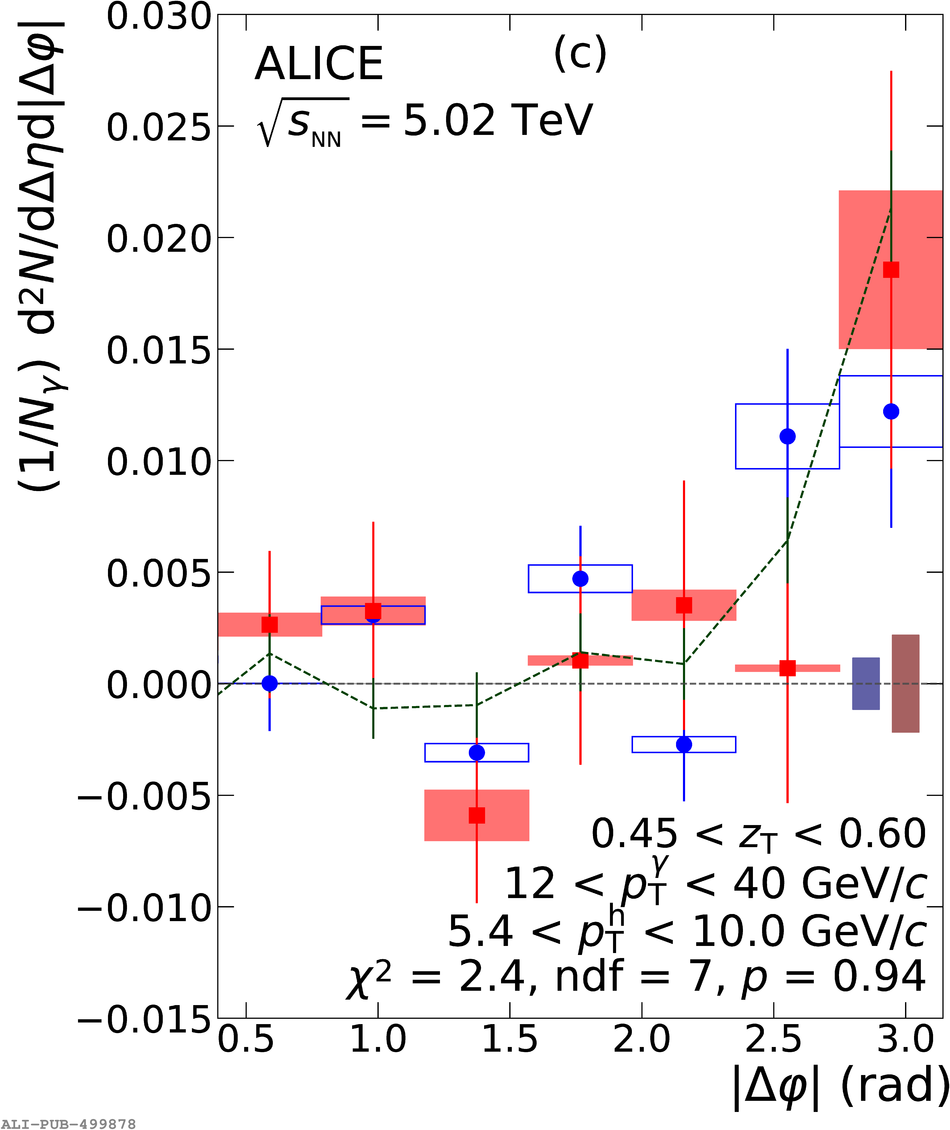# Figure 4

 $\gammaiso$--hadron correlation functions for pp (red) and \pPb (blue) data at $\sqrt{s_\mathrm{NN}}$ = 5.02 TeV as measured by the ALICE detector. The different panels represent three different \zt bins. The correlation functions are projected over the range \$|\Delta\eta|